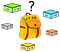# Intro to dynamic programming knapsack problems

Dynamic programming problems are frequently asked in a tech interview. For me, dynamic programming problems are challenging to understand and to solve. Recently, I see a post demonstrating that dynamic programming problems can be categorized. One of the categories is the Knapsack problem. Today, I'll introduce what’s knapsack problem and its related topics.

## Definition

The knapsack problem is a problem in combinatorial optimization: Given a set of items, each with a weight and a value, determine the number of each item to include in a collection so that the total weight is less than or equal to a given limit and the total value is as large as possible.This file is licensed under the Creative Commons Attribution-Share Alike 2.5 Generic license.

That’s said if we have items with the following weights and values, what’s the maximum value we can steal with the total weight that doesn’t exceed the maximum weight.

## The Brute-Force solution

When evaluating the maximum values to get, we need to consider all subsets of the given array. For each subset, we also need to make sure the total weight doesn’t exceed the max weight. The intuitive process would be: start from item 1, two consequences are a)take it; b)not take it. Followed by item 2, consequences a and b both have another two consequences: c)take item 2; d)not take item 2. …Until we met the last item in the array. The following graph visualizes this process.

As we can see from the graph, there are `2^n` combinations we need to consider, `n` is the number of items. Among these combinations, we find out that the maximum value we can get is \$15. The running time for the brute-force solution is `O(2^n)`. Time complexity is `O(1)`.

Code:

`class Solution:    def knapsack(self, values, weights, maxWeightConstraint):        self.res = 0        def backtrack(values, weights, maxWeightConstraint, currWeight, currVal):            if currWeight > maxWeightConstraint:                return            else:                self.res = max(self.res, currVal)            for i in range(len(weights)):                backtrack(values[i+1:], weights[i+1:], maxWeightConstraint, currWeight+weights[i], currVal+values[i])        backtrack(values, weights, maxWeightConstraint, 0, 0)        return self.res`

## Dynamic programming solution

Dynamic programming algorithm performs well in reducing the running time by adding extra space to memorize the results of different combinations. How to solve the knapsack problem using this algorithm? Let’s take several steps to think about the process:

Step 1: break down the problem into multiple subproblems

To determine the maximum values we can get from the given items and weights, let’s start with a smaller scale. At the weight `j,j in range[0,max weight]`and item `i` , what’s the maximum value we can get at the current state?

Step 2: determine the initial state

The best data structure to store the maximum value we get for `item[i]weight[j]` is a 2D matrix. The row number is the (total item count + 1), and the column number is (max weight + 1). The reason we increment the size by 1 is to determine the initial state: when the item is `None` and the weight is 0, the value we get is 0.

Step 3: solve the subproblem

If we’re only given one item and its weight doesn’t exceed the maximum weight, obviously the result is its value. If we gradually increase the size of given items, how to determine the items to pick? For each item, there’re two scenarios: pick it or not pick it. The subproblem becomes: if we pick the current item, `item[i]weight[j] = item[i-1]weight[j-wi]+vi` , if we don’t pick the current item, `item[i]weight[j] = item[i-1]weight[j]` . We need to find the maximum value among these two, as the result, `item[i]weight[j] = max(item[i-1]weight[j-wi]+vi, item[i-1]weight[j])` .

Code:

`def knapsack(values, weights, maxWeightConstraint):    row, col = len(values)+1, maxWeightConstraint+1    dp = [[0 for _ in range(col)] for _ in range(row)]    for i in range(1, row):        for j in range(1, col):            if j >= weights[i-1]:                dp[i][j] = max(dp[i-1][j], dp[i-1][j-weights[i-1]]+values[i-1])    return dp[row-1][col-1]`

Compare with the brute force solution, the dynamic programming approach greatly reduces the running time to `O(n*m)`, where n is the size of items, m is the max weight. However, since it introduces a matrix with size(n*m), the space complexity increases to `O(m*n)`.

## Related topics

Many dynamic programming problems can be solved by implementing the knapsack’s algorithm. Here’s the list of similar problems:

Thanks for reading!

## Reference

on the way to become a programmer

## More from Wangyy

on the way to become a programmer